# Index of Hydrogen Deficiency (IHD)

$$\newcommand{\vecs}{\overset { \rightharpoonup} {\mathbf{#1}} }$$ $$\newcommand{\vecd}{\overset{-\!-\!\rightharpoonup}{\vphantom{a}\smash {#1}}}$$$$\newcommand{\id}{\mathrm{id}}$$ $$\newcommand{\Span}{\mathrm{span}}$$ $$\newcommand{\kernel}{\mathrm{null}\,}$$ $$\newcommand{\range}{\mathrm{range}\,}$$ $$\newcommand{\RealPart}{\mathrm{Re}}$$ $$\newcommand{\ImaginaryPart}{\mathrm{Im}}$$ $$\newcommand{\Argument}{\mathrm{Arg}}$$ $$\newcommand{\norm}{\| #1 \|}$$ $$\newcommand{\inner}{\langle #1, #2 \rangle}$$ $$\newcommand{\Span}{\mathrm{span}}$$ $$\newcommand{\id}{\mathrm{id}}$$ $$\newcommand{\Span}{\mathrm{span}}$$ $$\newcommand{\kernel}{\mathrm{null}\,}$$ $$\newcommand{\range}{\mathrm{range}\,}$$ $$\newcommand{\RealPart}{\mathrm{Re}}$$ $$\newcommand{\ImaginaryPart}{\mathrm{Im}}$$ $$\newcommand{\Argument}{\mathrm{Arg}}$$ $$\newcommand{\norm}{\| #1 \|}$$ $$\newcommand{\inner}{\langle #1, #2 \rangle}$$ $$\newcommand{\Span}{\mathrm{span}}$$$$\newcommand{\AA}{\unicode[.8,0]{x212B}}$$

There is no simple way of predicting how many isomers a given molecular formula will yield, (it can range from one to many). Structures are different if they cannot be superimposed upon one another. Keep in mind that there is rotation about all single bonds not involved in a ring, but not about double bonds. Because all of the formulas that you will be dealing with are based on the $$C$$ atom, it may be useful to review the ways that $$C$$ can bond to itself and to other atoms. We will limit ourselves, for now, to the $$C$$ atom with four bonds. Below are the possible combinations of C having a total of four bonds.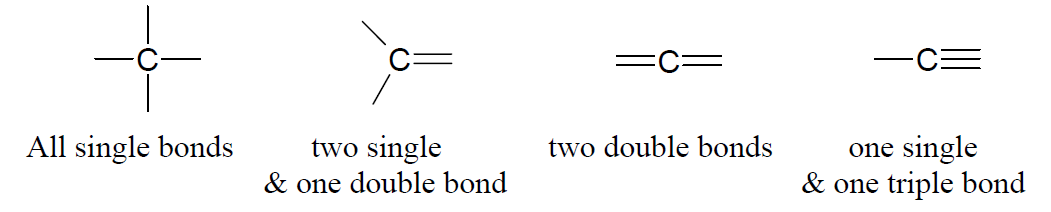In a hydrocarbon where all the C atoms have only single bonds and no rings are involved, the compound would have the maximum number of H atoms. If any of the bonds are replaced with double or triple bonds, or if rings are involved, there would be a “deficiency” of H atoms. By calculating the index of hydrogen deficiency (IHD), we can tell from the molecular formula whether and how many multiple bonds and rings are involved. IHD is also called the Degree of Unstaturation. This will help cut down the possibilities one has to consider in trying to come up with all the isomers of a given formula.

Here is a summary of how the index of hydrogen deficiency (IHD) works.

• A double bond and ring each counts as one IHD.
• A triple bond counts as two IHD.

Hydrocarbons ($$C_xH_y$$):

$IHD = \dfrac{2x + 2 - y}{2}$

(where $$x$$ and $$y$$ stand for # of C and H respectively.)

Example 1

IHD for $$C_2H_4$$ is

$\dfrac{2(2) + 2 - 4}{2} = 1$

This means it can have either one double bond or one ring, but it cannot have a triple bond. Since you cannot form a ring with only two C’s, it must have a double bond.

Example 2

IHD for $$C_4H_6$$ is

$\dfrac{2(4) + 2 - 6}{2} = 2$

This means it can have either one double bond and a ring such as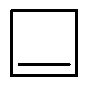or two double bonds such as CH2=CH−CH=CH2 or CH2=C=CH−CH3 or two rings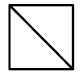, or one triple bond, such as CH3C≡CCH3.

## Compounds Containing Elements Other than C and H

O and S atoms do not affect the IHD.

• Halogens (F, Cl, Br, I) are treated like H atoms (CH2Cl2 has the same IHD as CH4).
• For each N, add one to the number of C and one to the number H (CH5N is treated as C2H6. CH4N2O is treated as C3H6 by adding 2 to # of C and 2 to # of H).

Do not forget that when double bonds and rings are involved, geometric isomers are possible.

### Practice problems

Calculate the IHD for each of the following and see whether it corresponds to the structure shown. (Obviously it should!) Don’t peek until you’ve worked it out yourself, but answers are provided at the bottom.

a)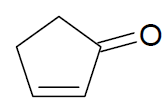b) CH3CHCHCH2CHCH2

c)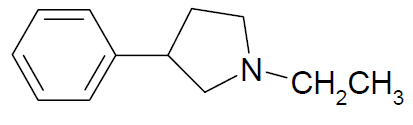d)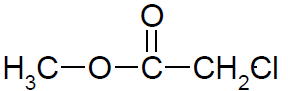e) CH3C≡CCOCH3